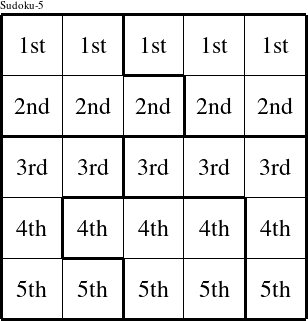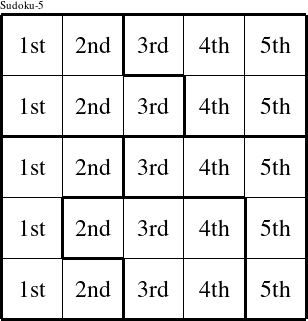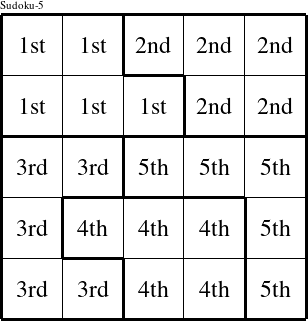#### Printable version

Detailed instructions for working a group puzzle of type 'Shawn'

This is a signature puzzle named 'Shawn'

The 5 elements in each group of this type of puzzle are: S, H, A, W, and N.

Every group in the puzzle must contain exactly this set of elements.

####In a Shawn puzzle, each row is a group numbered as shown in the figure above.

####In a Shawn puzzle, each column is a group numbered as shown in the figure above.

####In a Shawn puzzle, each pentomino is a group numbered as shown in the figure above.